##### Pre-Calculus: 1001 Practice Problems For Dummies (+ Free Online Practice)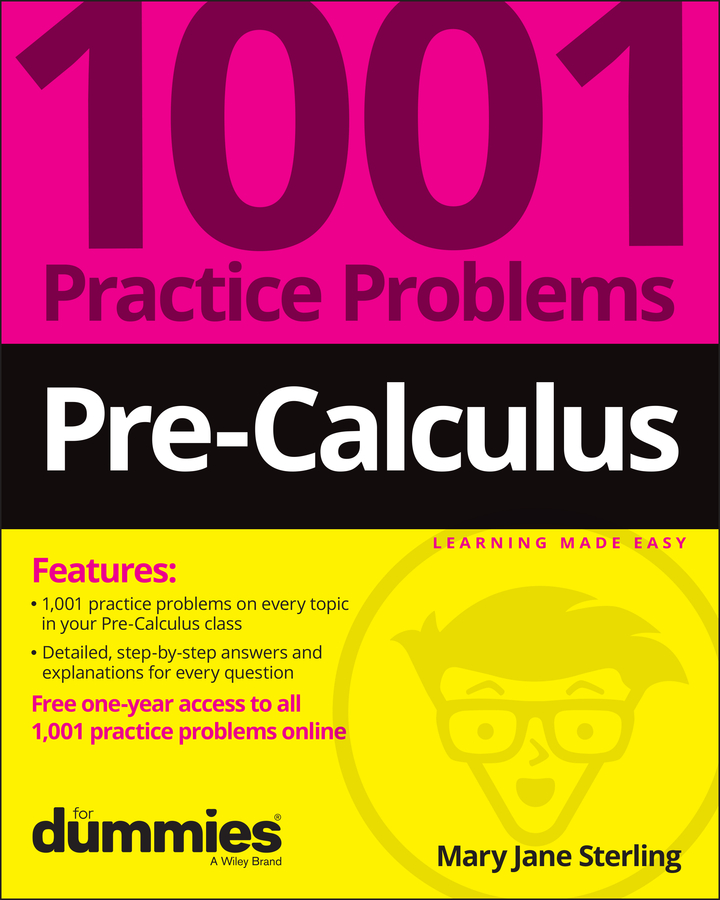The period of the parent graphs of sine and cosine is 2 multiplied by pi, which is once around the unit circle. Sometimes in trigonometry, the variable x, not the function, gets multiplied by a constant. This action affects the period of the trig function graph.

For example, f(x) = sin 2x makes the graph repeat itself twice in the same amount of time; in other words, the graph moves twice as fast. Think of it like fast-forwarding a DVD. This figure shows function graphs with various period changes.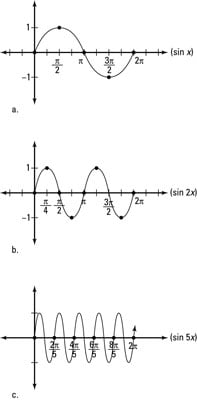Creating period changes on function graphs.

To find the period of f(x) = sin 2x,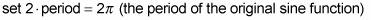and solve for the period. In this case,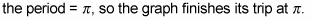Each period of the graph finishes at twice the speed.

You can make the graph of a trig function move faster or slower with different constants:

• Positive values of period greater than 1 make the graph repeat itself more and more frequently.

You see this rule in the example of f(x).

• Fraction values between 0 and 1 make the graph repeat itself less frequently.

For example, if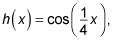you can find its period by setting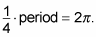Solving for period gets you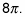Before, the graph finished at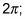now it waits to finish at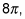which slows it down by 1/4.

You can have a negative constant multiplying the period. A negative constant affects how fast the graph moves, but in the opposite direction of the positive constant. For example, say p(x) = sin(3x) and q(x) = sin(–3x). The period of p(x) is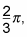whereas the period of q(x) is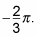The graph of p(x) moves to the right of the y-axis, and the graph of q(x) moves to the left. The figure illustrates this point clearly. Keep in mind that these graphs represent only one period of the function. The graph actually repeats itself in both directions infinitely many times.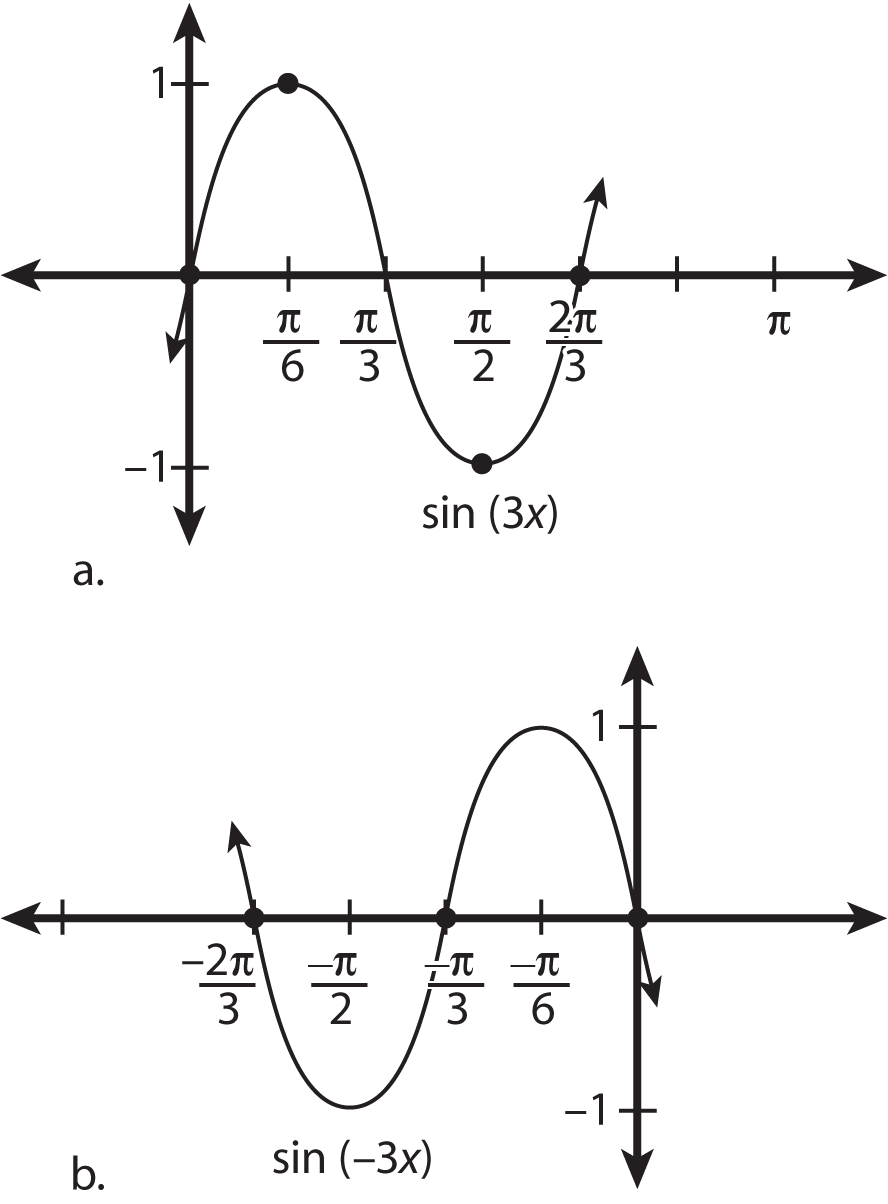Graphs with negative periods move to the opposite side of the y-axis.

Don't confuse amplitude and period when graphing trig functions. For example, f(x) = 2 sin x and g(x) = sin 2x affect the graph differently: f(x) = 2 sin x makes it taller, and g(x) = sin 2x makes it move faster.